# Splay学习笔记

6 篇文章 0 订阅

struct tree
{
int val,sz,cnt;//val为值 sz为子树大小，cnt为"有多少这个值"
//cnt:假如出现了两个一模一样的值，只需要让cnt+1就可以了，cnt是数目
int s,f;//s为左儿子 s为右儿子 f为父亲
};

bool son(int x) //返回x是左儿子还是右儿子，如果为左儿子，返回0，右儿子则返回1
{
return a[a[x].f].s == x;
}

void rejs(int x)    //重新计算以x为根，子树的大小，子树大小等于左子树大小加右子树大小(好吧我承认我英语拙计)
{
a[x].sz = a[a[x].s].sz + a[a[x].s].sz + a[x].cnt;
}

void point(int x,int y,bool z)  //在x下面插入y，y是x的z儿子(z为0则左儿子，z为1为右儿子)
{
a[x].s[z] = y;
a[y].f = x;
}

Splay最重要的一个操作就是旋转(rotate)，如下，(图片为1600x900，可直接作为桌面)：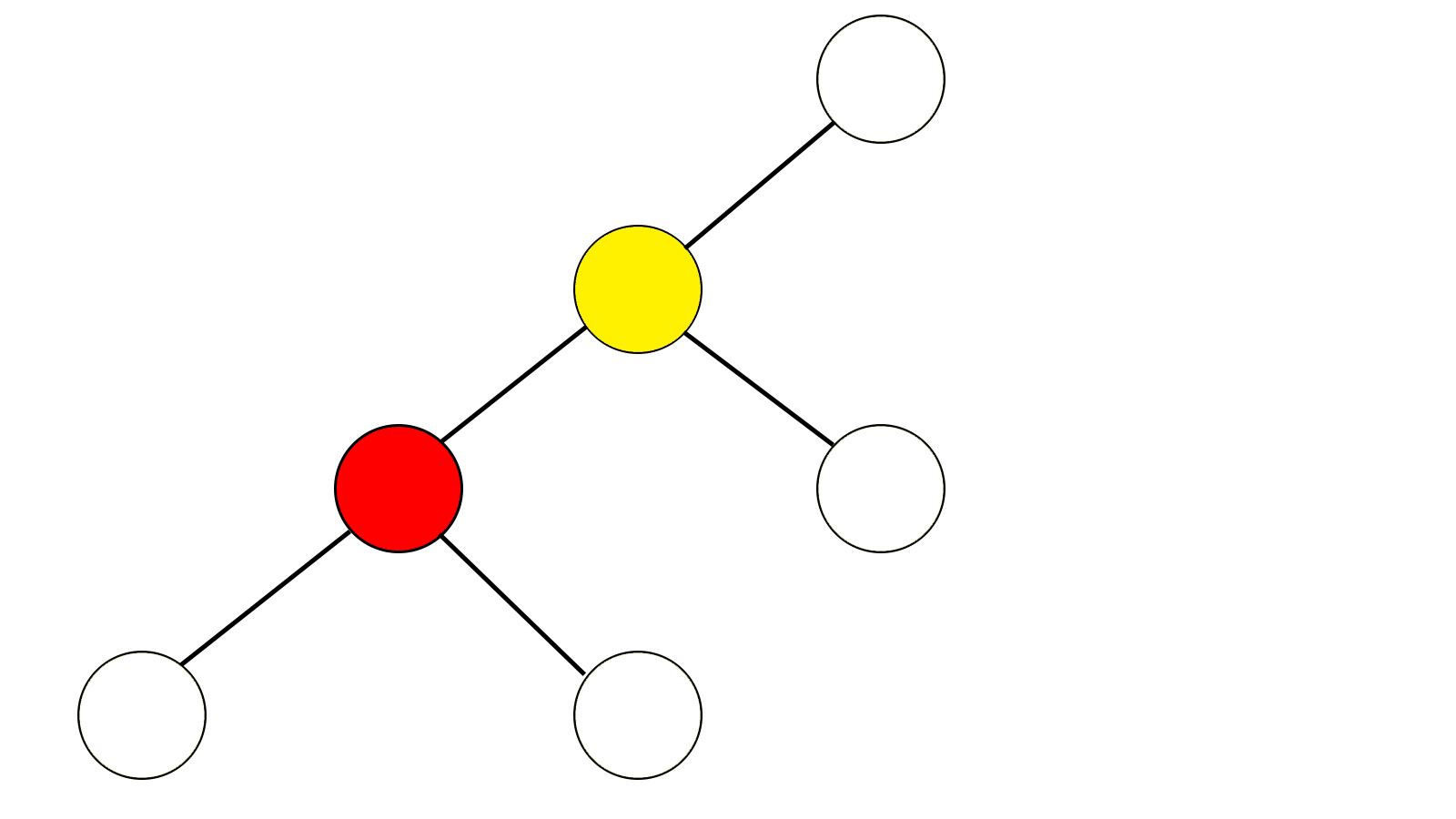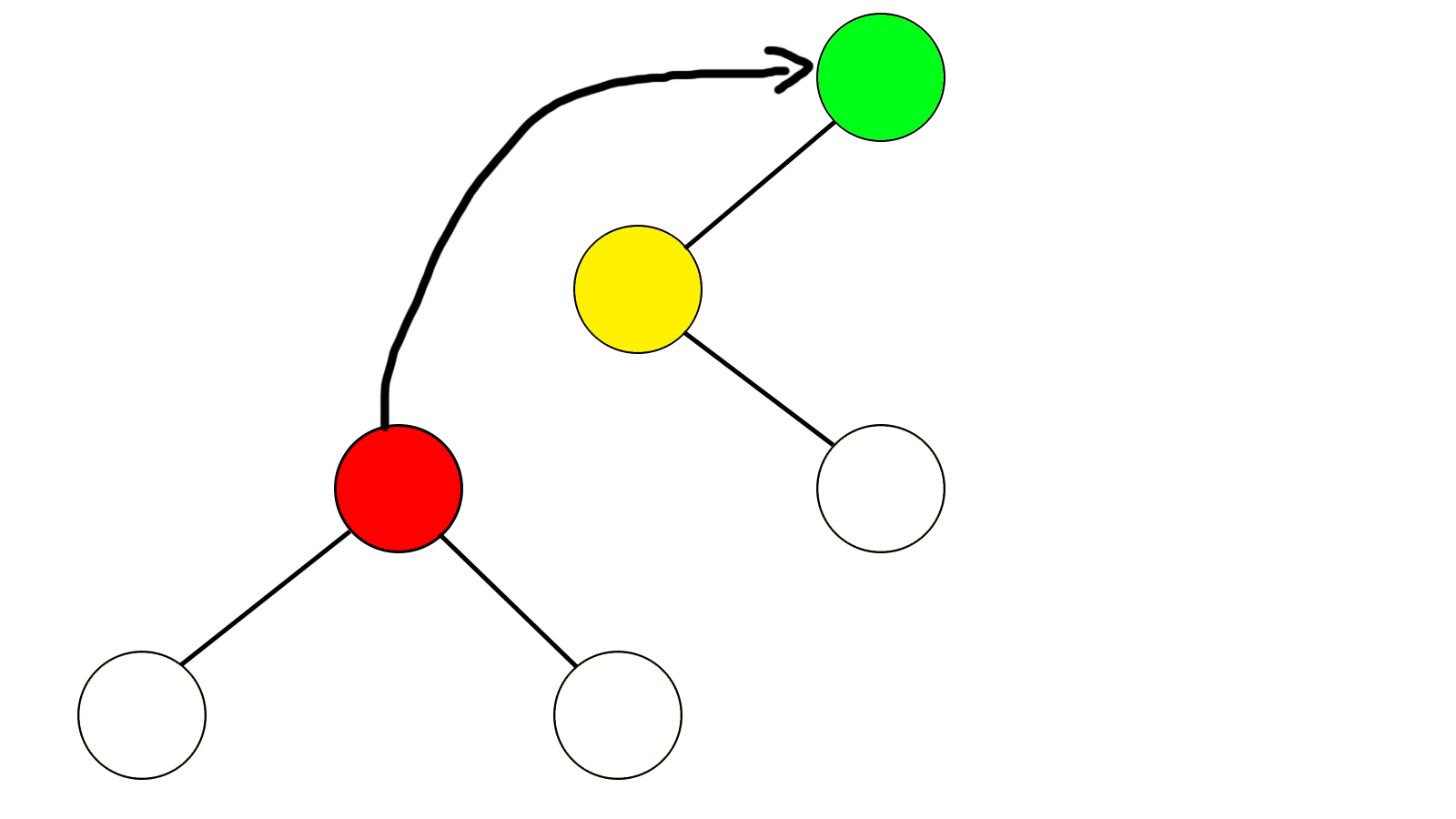bool型变量son(黄)代表黄色节点是左儿子还是右儿子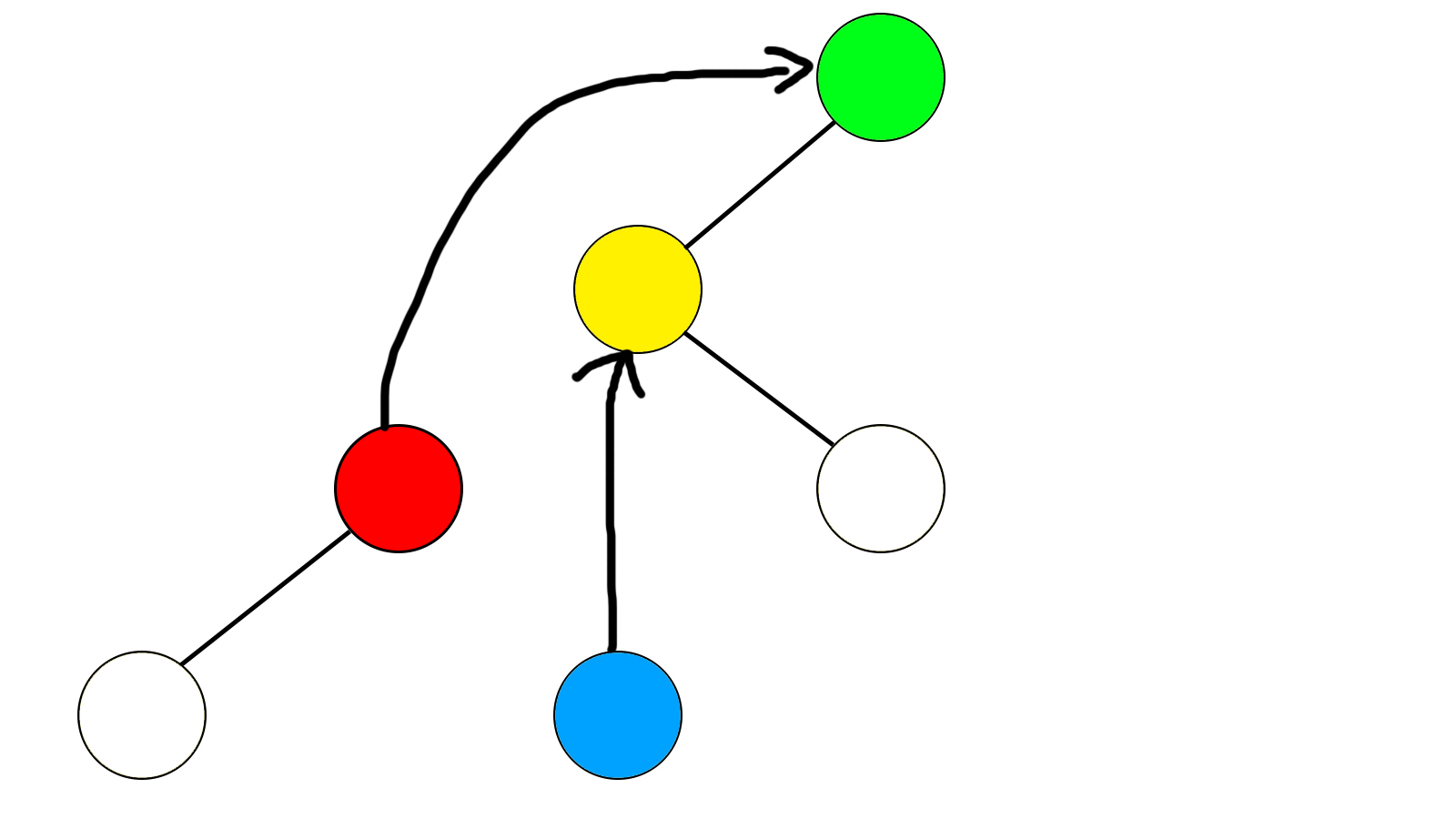bool型变量son(红)代表红色节点是左儿子还是右儿子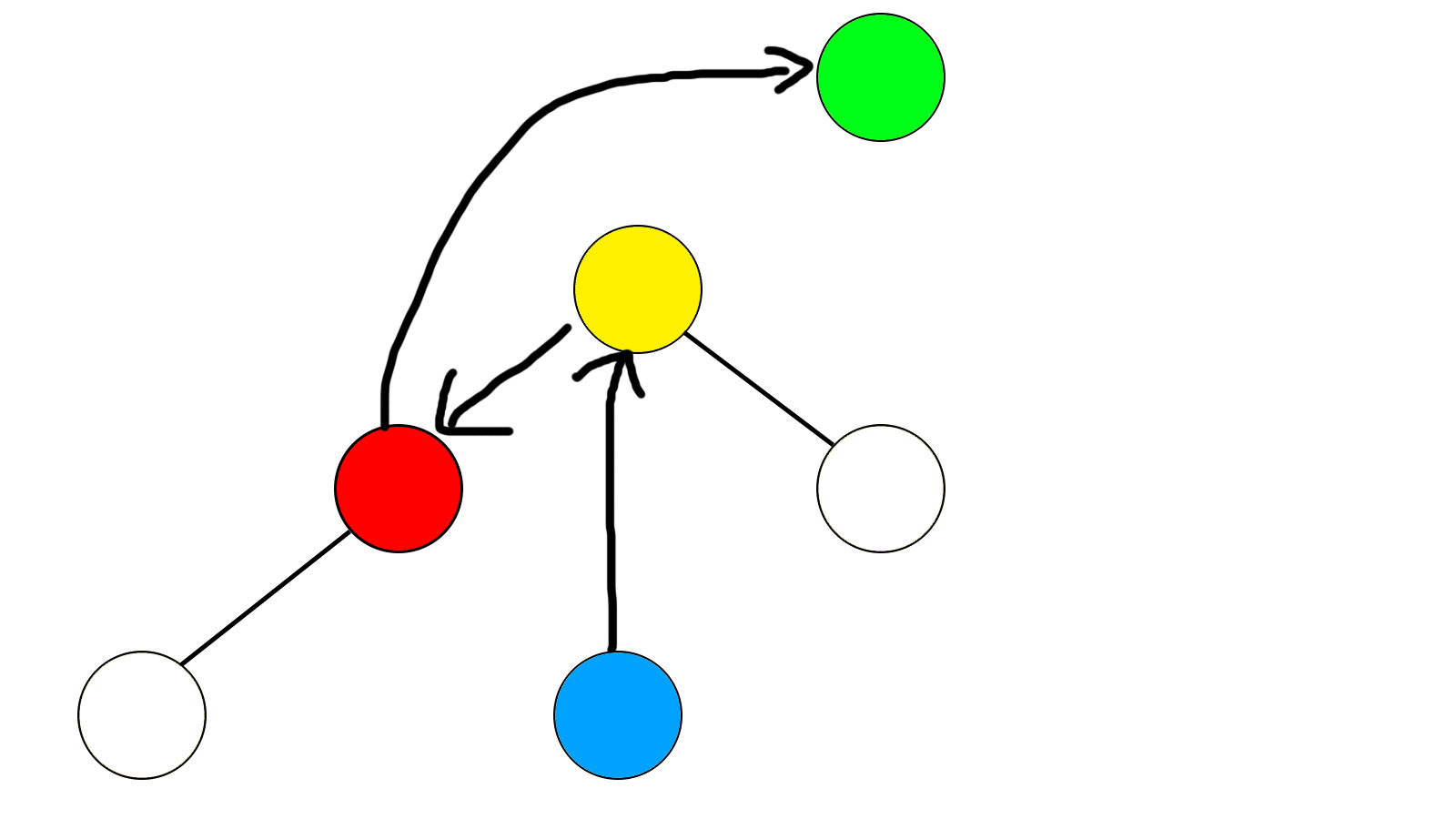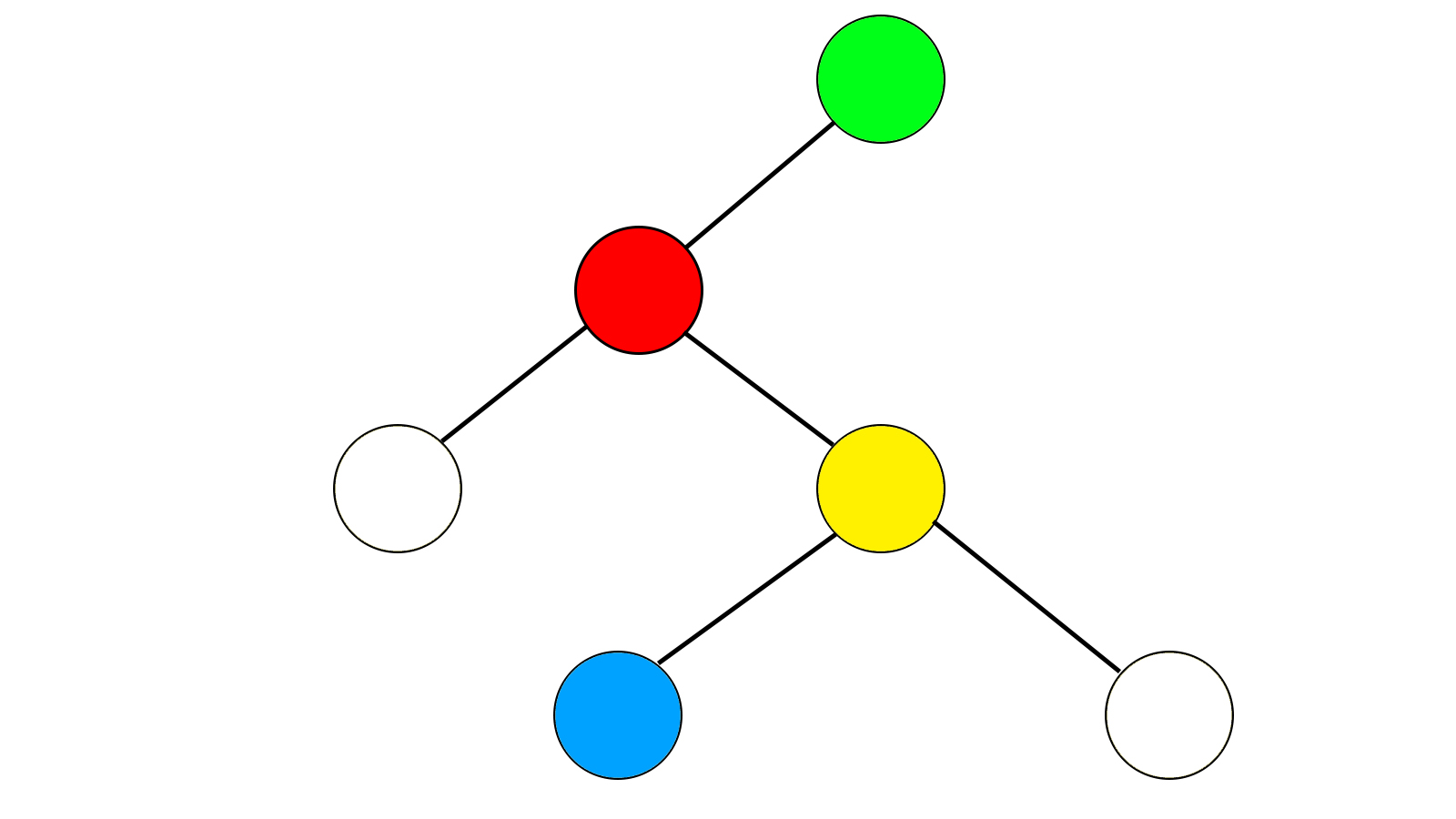(我再也不用PS画这玩意了……)

void rot(int x)
{
int p = a[x].f;
bool d = son(x);
point(a[p].f,x,son(p));
point(p,a[x].s[d^1],d);
point(x,p,d^1);
rejs(p);
rejs(x);
if(a[x].f == 0)
root = x;//root是根节点的编号
}

splay操作的目的是将一个点旋转到根节点，这个操作是这样的：
1.如果这个点是根节点，那么你可以直接退出了……
2.如果这个点的父亲是根节点，那么就直接把这个点rotate上去……
3.如果上述两条均不满足，那么分类讨论：
(1)设son(x)为这个和父亲节点的关系，son(f)为父亲节点和爷爷节点的关系
(2)如果两个关系相同(均为左儿子或均为右儿子)，那么就先rotate父亲节点，然后rotate这个节点
(3)如果两个关系不同(一个是左儿子一个是右儿子)，那么就把这个节点连续rotate两次

void splay(int x)
{
while(a[x].f != 0){
if(a[a[x].f].f == 0)
rot(x);
else
{
if(son(x) == son(a[x].f)){
rot(a[x].f);
rot(x);
}
else{
rot(x);
rot(x);
}
}
}
}

void ins(int x)
{
int w = root,f = 0;
int p = findn(x);
if(p)//如果这个值已经存在，那么就直接给这个节点+1吧
{
a[p].cnt ++;
while(p != root)
{
rejs(p);
p = a[p].f;
}
rejs(root);
return ;
}
while(w)
{
f = w;
if(x < a[w].val)
w = a[w].s;
else
w = a[w].s;
}
//这是前半部分，和普通的二叉查找树插入方法一样，我承认我打的很丑……
a[++tot].val = x;
a[tot].cnt = 1;//新建节点，标号为tot
if(f == 0)//如果这个点是根节点的话，直接插入即可……
{
root = tot;
rejs(root);
return ;
}
//否则插入这个节点并spaly到根节点
if(x < a[f].val)
point(f,tot,0);
else
point(f,tot,1);
splay(tot);
}

x的前驱是指小于x且最大的节点，后继就是大于x且最小的节点

int near(int x,bool d)
{
if(a[x].s[d] != 0){
int p = a[x].s[d];
while(a[p].s[d^1])
p = a[p].s[d^1];
return p;
}
else if(son(x) == d^1)
return a[x].f;
else{
int p = a[x].f;
while(son(p) == d){
if(p == root)
return 0;
p = a[p].f;
}
return a[p].f;
}
}

x成为了x前驱的右子树！直接砍掉这个右子树就ok啦~(如果删除区间也可以这样做，splay区间左边界的前驱，splay区间右边界的后继，整个区间就变成了区间左界前驱的右子树~(≧▽≦)/~)

void cle(int x)//cle操作是清除一个点，没有也无所谓吧
{
a[a[x].f].s[son(x)] = 0;
a[x].val = 0;
a[x].sz = 0;
rejs(a[x].f);
a[x].f = 0;
}

void del(int x)
{
int p = near(x,0);
int q = near(x,1);
if(!p || !q)
{
splay(x);
if(p == 0)
{
root = a[x].s;
a[a[x].s].f = 0;
}
else
{
root = a[x].s;
a[a[x].s].f = 0;
}
return ;
}
splay(p);
splay(q);
rot(p);
cle(x);
}

11-14
06-1216010-28104
05-272414
05-127095
07-312898
08-122555
08-262069
08-262万+
11-022万+
11-14428
03-0619
02-032万+
03-17271
04-26580
12-17547
02-1135
03-05971
02-281万+
02-01183点击重新获取扫码支付余额充值